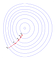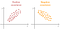# Linear Regression :

Linear regression is one of the simplest machine learning algorithm which belongs to Supervised learning. Mathematically it can be represented by the equation Y = m*x + b

Example : Here Y = Price, X = Area

# Visualizing Gradient Descent and its types

Gradient descent is one of the most important optimization technique. The basic idea behind gradient descent is to reduce the error by increasing the learning rate slowly and by updating the parameters. So the main aim here is to reach the global minima and minimize the error as much as possiblePicture taken from Wikipedia

The above picture shows how the gradient descent technique tries to minimize the error by increasing the learning rate slowly and reaching to that global minima. There are 3 types of gradient descent algorithms

# Batch Gradient Descent :

It is a technique where the model goes through all the data points in every…

# Covariance :

Covariance is one of the most used topic in data analysis or data pre processing. It is used to Quantify the relationship between features in a particular dataset. In simple words, it is used to understand the relationship between 2 or more different columns from a dataset.Photo taken from Google Cloud

Math behind the covariance is similar to that of variance which is quite interesting. As we know that covariance is used for 2 variables and we can denote it as Covariance(x,y). Here Covariance(x,x) is equal to that of variance(x). This is how both variance and covariance are related to each other.

After all…

# The 2 Most underrated techniques in data science - Grid search CV, Randomized search CV?

Any model in machine learning should be trained and tested before deploying the model. The simplest way is the traditional “Train_Test_split” method. It trains some portion of the data say 70% or 80%, It will test the remaining data. As all the data is not trained and tested there is a probability of missing some features in the data.

We can use K fold cross validation which makes sure that all the data is trained and tested. But it is a manual method, you can use some loops while coding, to perform this technique.

Is there any technique which trains…## Sjatin

Self motivated writer, Loves reading anything. Interested in exploring new technologies like DL, ML, AI. Writing is my passion and I would never leave it